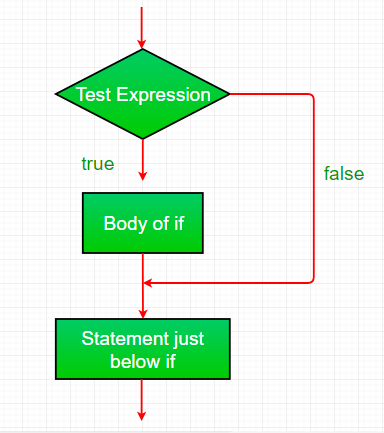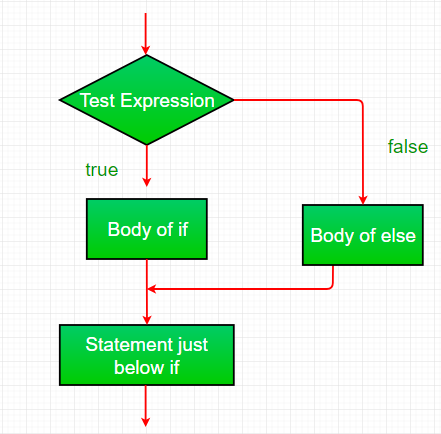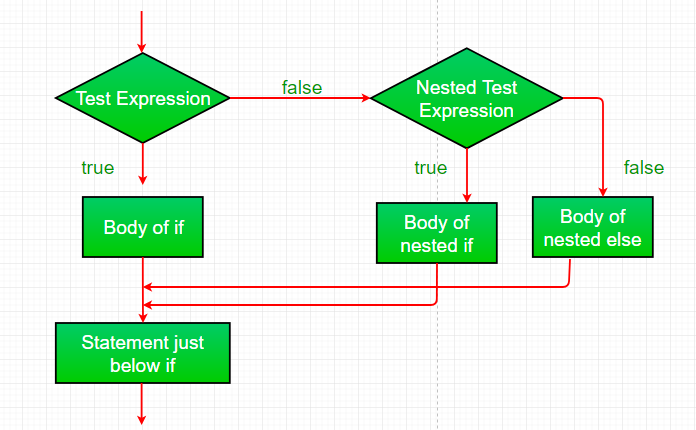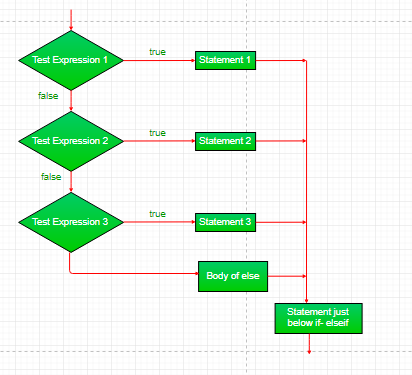GFG App
Open AppBrowser
Continue

The if-else or conditional statement will perform some action for a specific condition. If the condition meets then a particular block of action will be executed otherwise it will execute another block of action that satisfies that particular condition. Such control statements are used to cause the flow of execution to advance and branch based on changes to the state of a program.

Example: This example describes the if-statement in Javascript.

## Javascript

 ``

Output:

`I am Not in if`

JavaScript’s conditional statements:

• if
• if-else
• nested-if

We will understand each conditional statement, its syntax, flowchart, and examples. Please refer to the Switch Case in JavaScript article to understand the switch case. Let’s begin with the if-statement.

JavaScript if-statement: It is a conditional statement used to decide whether a certain statement or block of statements will be executed or not i.e if a certain condition is true then a block of statement is executed otherwise not.

Syntax:

```if(condition)
{
// Statements to execute if
// condition is true
}```

The if statement accepts boolean values – if the value is true then it will execute the block of statements under it. If we do not provide the curly braces ‘{‘ and ‘}’ after if( condition ) then by default if statement considers the immediate one statement to be inside its block. For example,

```if(condition)
statement1;
statement2;

// Here if the condition is true, if block
// will consider only statement1 to be inside
// its block.```

Flow chart:if-condition statement

Example: Here is a simple example demonstrating the if statement.

## Javascript

 ``

JavaScript if-else statement: The if statement alone tells us that if a condition is true it will execute a block of statements and if the condition is false it won’t. But what if we want to do something else if the condition is false? Here comes the else statement. We can use the else statement with the if statement to execute a block of code when the condition is false.

Syntax:

```if (condition)
{
// Executes this block if
// condition is true
}
else
{
// Executes this block if
// condition is false
}```

Flow chart:if-else statement

Example: This example describes the if-else statement in Javascript.

## JavaScript

 ``

Output:

`i is less than 15`

JavaScript nested-if statement: JavaScript allows us to nest if statements within if statements. i.e, we can place an if statement inside another if statement. A nested if is an if statement that is the target of another if or else.

Syntax:

```if (condition1)
{
// Executes when condition1 is true
if (condition2)
{
// Executes when condition2 is true
}
}```

Flow chart:nested-if statement

Example: This example describes the nested-if statement in Javascript.

## JavaScript

 ``

Output:

```i is smaller than 15
i is smaller than 12 too```

JavaScript if-else-if ladder statement: Here, a user can decide among multiple options.The if statements are executed from the top down. As soon as one of the conditions controlling the if is true, the statement associated with that if is executed, and the rest of the ladder is bypassed. If none of the conditions is true, then the final else statement will be executed.

Syntax:

```if (condition)
statement;
else if (condition)
statement;
.
.
else
statement;```

Flow chart:Example: This example describes the if-else-if ladder statement in Javascript.

## JavaScript

 ``

Output:

`i is 20`

Supported Browsers: